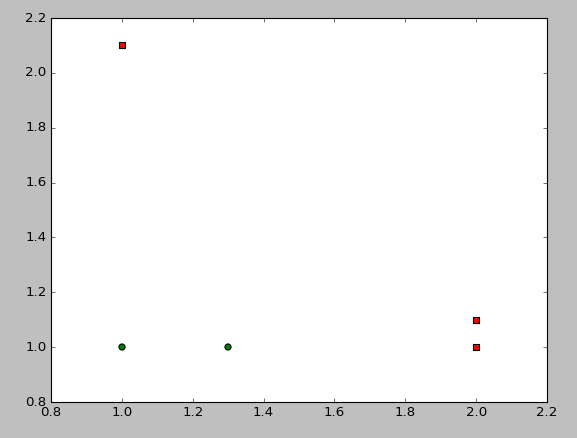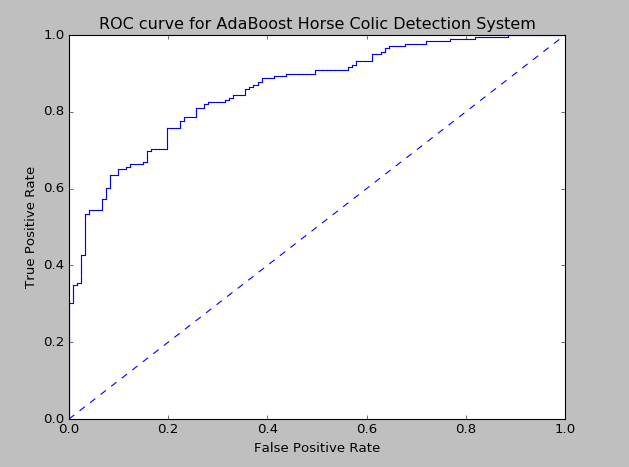----------------------------------------------------------------------------------

bagging方法

Bagging的策略【来源】：

- 从样本集中用Bootstrap采样选出n个样本

- 在所有属性上，对这n个样本建立分类器（CART or SVM or ...）

- 重复以上两步m次，i.e.build m个分类器（CART or SVM or ...）

- 将数据放在这m个分类器上跑，最后vote看到底分到哪一类

bagging方法是一种从原始数据及选择S次后得到S个新数据集的一种技术。新数据集和原数据集的大小相等。每个数据集都是通过在原始数据集中随机选择一个样本进行替换而得到的。（替换的方法可以是随机选择一个样本，再随机选择一个样本替换。）所以，新数据集中可以存在重复的值，同时，原始数据集的部分值在新数据集中不再出现。

bagging方法在S个数据集建好之后，将某个学习算法分别作用于每个数据集就得到了S个分类器。要对新数据进行分类时，就可以应用这S个分类器进行分类。选择分类器投票最多的类别作为分类结果。

-------来源--------------------------------------------------------------------------------------------------------------------------------------------------------------------------------------------------------------

• Random forest(Breiman1999):

- 从样本集中用Bootstrap采样选出n个样本，预建立CART

- 在树的每个节点上，从所有属性中随机选择k个属性，选择出一个最佳分割属性作为节点

- 重复以上两步m次，i.e.build m棵CART

- 这m个CART形成Random Forest

1. Bootstrap中的随机选择子样本
2. Random subspace的算法从属性集中随机选择k个属性，每个树节点分裂时，从这随机的k个属性，选择最优的

结果证明有时候Random Forest比Bagging还要好。今天微软的Kinect里面就采用了Random Forest，相关论文Real-time Human Pose Recognition in Parts from Single Depth Images是CVPR2011的best paper。
-------------------------------------------------------------------------------------------------------------------------------------------------------------------------------------------------

boosting方法

boosting方法是一种用来提高弱分类器算法准确度的方法。boosting方法是一种用来提高弱分类算法准确度的方法,这种方法通过构造一个预测函数系列,然后以一定的方式将他们组合成一个预测函数。他是一种框架算法,主要是通过对样本集的操作获得样本子集,然后用弱分类算法在样本子集上训练生成一系列的基分类器。

def loadSimpleData():
datMat=matrix([[1.,2.1],[2.,1.1],[1.3,1.],[1.,1.],[2.,1.]])
classLabel=[1.0,1.0,-1.0,-1.0,1.0]
return datMat,classLabeln=shape(dataMat)
xcord1=[];ycord1=[]
xcord2=[];ycord2=[]
for i in range(n):
if int(classLabel[i])== 1:
xcord1.append(dataMat[i,0]);ycord1.append(dataMat[i,1])
else:
xcord2.append(dataMat[i,0]);ycord2.append(dataMat[i,1])
fig=plt.figure()
ax.scatter(xcord1,ycord1,s=30,c='red',marker='s')
ax.scatter(xcord2,ycord2,s=30,c='green')
plt.show()

def stumpClassify(dataMatrix,dimen,threshVal,threshIneq):
#函数在数据与阈值比较进行分类
retArray=ones((shape(dataMatrix),1))
if threshIneq=='lt':
retArray[dataMatrix[:,dimen]<=threshVal]=-1.0
else:
retArray[dataMatrix[:,dimen]>threshVal]=-1.0
return retArray


def buildStump(dataArr,classLabels,D):
#找到数据集上最佳单层决策树，D是数据对应的权重向量
dataMatrix=mat(dataArr)
labelMat=mat(classLabels).T
m,n=shape(dataMatrix)
#获得数据行列数
numSteps=10.0
#设置步数
bestStump={}
bestClassEst=mat(zeros((m,1)))
#初始化最佳
minError=inf
for i in range(n):
#遍历每个特征维度
rangeMin=dataMatrix[:,i].min()
rangeMax=dataMatrix[:,i].max()
stepSize=(rangeMax-rangeMin)/numSteps
#根据该特征的最大最小值设置步长
for j in range(-1,int(numSteps)+1):
#循环在步长上进行遍历
for inequal in ['lt','gt']:
threshVal=(rangeMin+float(j)*stepSize)
#根据步长设置阈值
predictedVals=stumpClassify(dataMatrix,i,threshVal,inequal)
#按照阈值分类，最简单的分类方法
errArr=mat(ones((m,1)))
errArr[predictedVals==labelMat]=0
#统计错误个数
weightedError=D.T*errArr
#错误样本的加权求和
print("split: dim %d, thresh %.2f, thresh inequal:%s, the weighted error is %.3f" %(i,threshVal,inequal,weightedError))
if weightedError<minError:
minError=weightedError
bestClassEst=predictedVals.copy()
bestStump['dim']=i
bestStump['thresh']=threshVal
bestStump['ineq']=inequal
return bestStump,minError,bestClassEst

def adaBoostTrainDS(dataArr,classLabels,numIt=40):
weakClassArr=[]
m=shape(dataArr)
#获得数据个数
D=mat(ones((m,1))/m)
#初始化权重，平均值
aggClassEst=mat(zeros((m,1)))
for i in range(numIt):
bestStump,error,classEst=buildStump(dataArr,classLabels,D)
#选择弱分类器
print("D:",D.T)
alpha=float(0.5*log((1.0-error)/max(error,1e-16)))
#alpha是每个弱分类器对应的权重
#此处max(error,1e-16)是防止error为0导致溢出
bestStump['alpha']=alpha
weakClassArr.append(bestStump)
#把当前弱分类器加入到弱分类器数组里
print("classEst:",classEst.T)
expon=multiply(-1*alpha*mat(classLabels).T,classEst)
#根据是否被错分是不一样的，分对权重低、分错权重高。
D=multiply(D,exp(expon))
D=D/D.sum()
#更新权重向量D，D表示样本的权重，根据是否被错分会进行调整
aggClassEst+=alpha*classEst
#每个数据点的类别累计值
print("aggClassEst:",aggClassEst.T)
aggError=multiply(sign(aggClassEst)!=mat(classLabels).T,ones((m,1)))
#累加错误
errorRate=aggError.sum()/m
print("total error:",errorRate,"\n")
if errorRate==0.0:break
#如果错误率为0也停止循环
return weakClassArr,aggClassEst

def adaClassify(datToClass,classifierArr):
dataMatrix=mat(datToClass)
m=shape(dataMatrix)
aggClassEst=mat(zeros((m,1)))
#对应的结果
for i in range(len(classifierArr)):
classEst=stumpClassify(dataMatrix,classifierArr[i]['dim'],\
classifierArr[i]['thresh'],classifierArr[i]['ineq'])
#由分类函数可以得到每个弱分类器的分类结果
aggClassEst+=classifierArr[i]['alpha']*classEst
#将每个结果加权求和得到最终的分类结果
print(aggClassEst)
return sign(aggClassEst)

def loadDataSet(fileName):
#获得特征的维数
dataMat=[]
labelMat=[]
fr=open(fileName)
lineArr=[]
curLine=line.strip().split('\t')
for i in range(numFeat-1):
lineArr.append(float(curLine[i]))
dataMat.append(lineArr)
labelMat.append(float(curLine[-1]))
return dataMat,labelMat

def plotROC(predStrengths,classLabels):
import matplotlib.pyplot as plt
cur=(1.0,1.0)
ySum=0.0
numPosClas=sum(array(classLabels)==1.0)
#统计真正例个数
yStep=1/float(numPosClas)
#1/正例个数为Y轴步长
xStep=1/float(len(classLabels)-numPosClas)
#1/反例个数为X轴步长
sortedIndicies=predStrengths.argsort()
#argsort返回的是从小到大排列后的索引值
fig=plt.figure()
fig.clf()
ax=plt.subplot(111)
for index in sortedIndicies.tolist():
if classLabels[index]==1.0:
delX=0
delY=yStep
else:
delX=xStep
delY=0
ySum+=cur
#划线时如果是正例，y轴改变，如果是反例，x轴改变。
ax.plot([cur,cur-delX],[cur,cur-delY],c='b')
#plot([x1,x2],[y1,y2])表示从(x1,y1)画到(x2,y2)
cur=(cur-delX,cur-delY)
#从右往左画
ax.plot([0,1],[0,1],'b--')
plt.xlabel('False Positive Rate')
plt.ylabel('True Positive Rate')
plt.title('ROC curve for AdaBoost Horse Colic Detection System')
ax.axis([0,1,0,1])
plt.show()
print('the Area Under the Curve is :',ySum*xStep)

TP和TN比较简单，T表示TRUE就是判断正确的意思。

FP+TN等同于数据集里真实的反例个数。TP+FN表示数据集里真实的正例个数。